8th Grade Math Home > Teacher Resources > Activities > Cylinder: Student Worksheet

 Cylinder: Student Worksheet Name: ____________________________________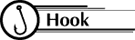If you compare three different cylinders, how can you tell which one will have the greatest volume?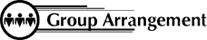Students work in individually or in pairs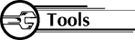3 sheets of paper with rectangles A, B, and C rulers tape scissorsCut out the rectangles shown on the attached pages. Tape them together on the sides indicated to form cylinders.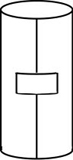Answer the following questions: If the cylinders had tops, what would be the diameter of each top? What would be the radius of each top? What would be the area of each top? Round to the nearest tenth. For the area of the tops, find A : B and A : C. Measure the heights of the three cylinders. For the heights, find: A : B ____________ A : C ____________ Predict the ratio of the volumes of the following cylinders: A and B __________ A and C __________ Find the volume of each cylinder. For the volumes, find: A : B ___________ A : C ___________ Were your predictions in Question 4 correct? Summarize: If the height of two cylinders is the same and the radii are in ratio of __?__, then their volumes are in the ratio of __?__. If the radius of two cylinders is the same and the heights are in ratio of __?__, then their volumes are in the ratio of __?__.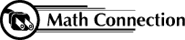As a result of this activity, students will be able to model how the changes of a figure in such dimensions as length, width, height, or radius affect other measurements such as perimeter, area, surface area, or volume.

 8th Grade Math Home > Teacher Resources > Activities > Cylinder: Student Worksheet